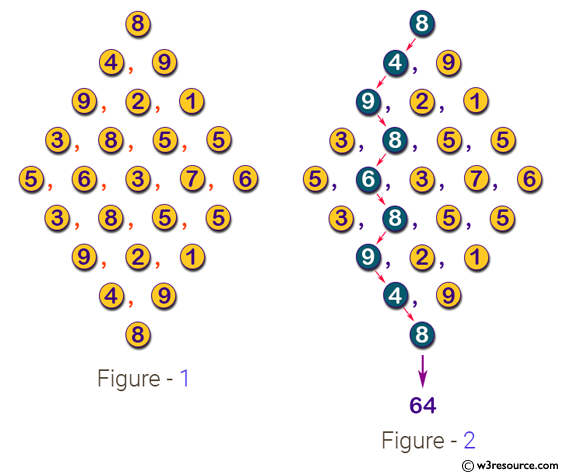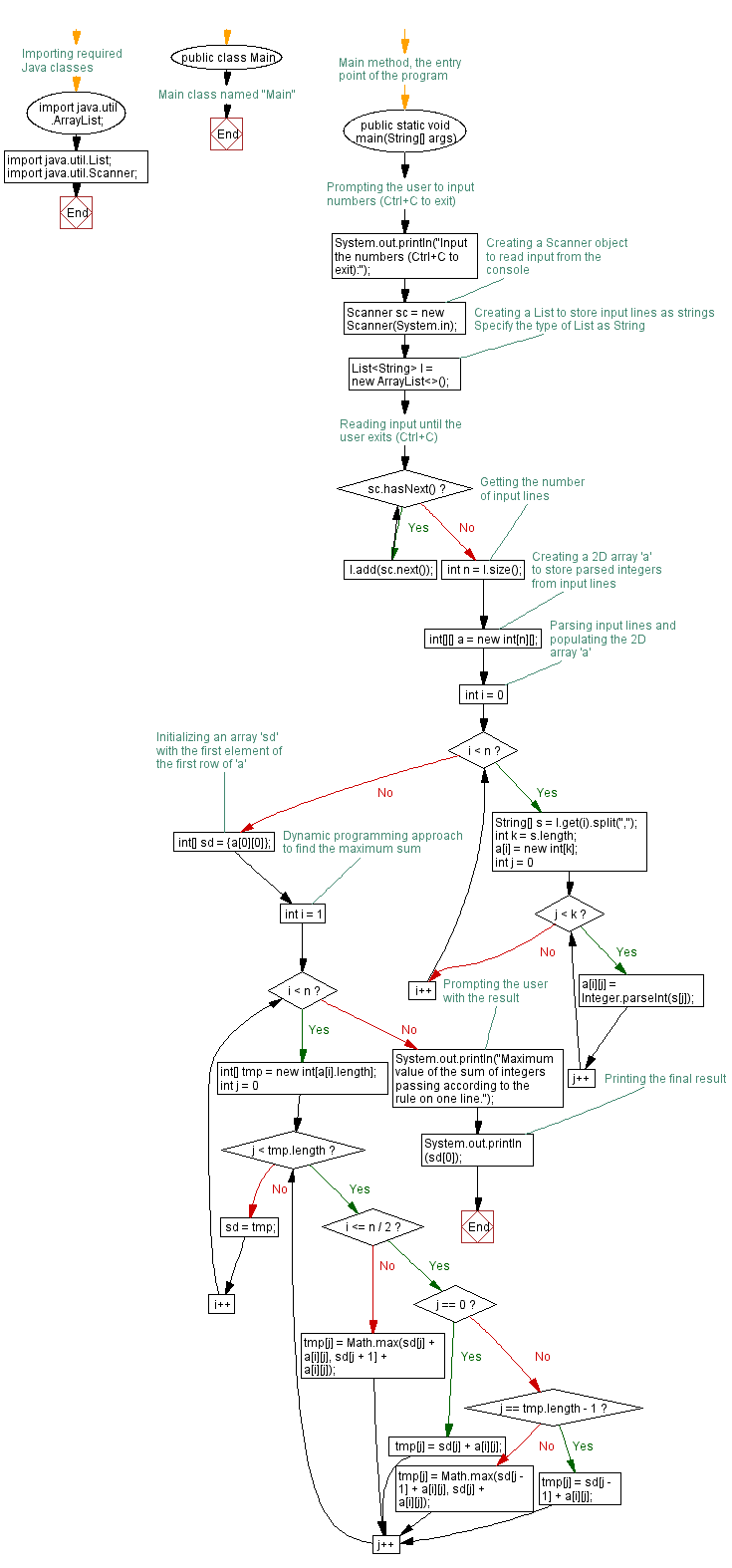# Java Exercises: Compute the maximum value of the sum of the passing integers

## Java Basic: Exercise-240 with Solution

Arrange integers (0 to 99) as narrow hilltop, as illustrated in Figure 1. Reading such data representing huge, when starting from the top and proceeding according to the next rule to the bottom. .
Write a Java program that compute the maximum value of the sum of the passing integers.

Input:
A series of integers separated by commas are given in diamonds. No spaces are included in each line. The input example corresponds to Figure 1. The number of lines of data is less than 100 lines.
Output: The maximum value of the sum of integers passing according to the rule on one line.

Pictorial Presentation:Sample Solution:

Java Code:

`````` import java.util.ArrayList;
import java.util.List;
import java.util.Scanner;

public class Main{

public static void main(String[] args) {
System.out.println("Input the numbers (ctrl+c to exit):");
Scanner sc = new Scanner(System.in);
List<String> l = new ArrayList<String>();
int n = l.size();
int[][] a = new int[n][];
for(int i=0;i<n;i++){
String[] s = l.get(i).split(",");
int k = s.length;
a[i] = new int[k];
for(int j=0;j<k;j++)a[i][j]=Integer.parseInt(s[j]);
}
int[] sd = {a};
for(int i=1;i<n;i++){
int[] tmp = new int[a[i].length];
for(int j=0;j<tmp.length;j++){
if(i<=n/2){
if(j==0)tmp[j]=sd[j]+a[i][j];
else if(j==tmp.length-1)tmp[j]=sd[j-1]+a[i][j];
else tmp[j]=Math.max(sd[j-1]+a[i][j], sd[j]+a[i][j]);
}
else{
tmp[j] = Math.max(sd[j]+a[i][j], sd[j+1]+a[i][j]);
}
}
sd = tmp;
}
System.out.println("Maximum value of the sum of integers passing according to the rule on one line.");
System.out.println(sd);
}
}
```
```

Sample Output:

```Input the numbers (ctrl+c to exit):
8
4,9
9,2,1
3,8,5,5
5,6,3,7,6
3,8,5,5
9,2,1
4,9
8
Maximum value of the sum of integers passing according to the rule on one line.
64
```

Flowchart:Java Code Editor:

What is the difficulty level of this exercise?

﻿

## Java: Tips of the Day

Parsing dates:

```import java.io.*;
import java.util.*;
import java.text.*;

String s = "2001/09/23 14:39";

SimpleDateFormat formatter = new SimpleDateFormat ("yyyy/MM/dd H:mm");
Date d = formatter.parse(s, new ParsePosition(0));
```